# Excel array formulas, functions and constants - examples and guidelines

In this tutorial, you will learn what an Excel array formula is, how to enter it correctly in your worksheets, and how to use array constants and array functions.

Array formulas in Excel are an extremely powerful tool and one of the most difficult to master. A single array formula can perform multiple calculations and replace thousands of usual formulas. And still, 90% of users have never used array functions in their worksheets simply because they are scared to start learning them.

Indeed, array formulas one of the most confusing Excel features to learn. The aim of this tutorial is to make the learning curve as easy and smooth as possible.

## What is an array in Excel?

Before we start on array functions and formulas, let's figure out what the term "array" means. Essentially, an array is a collection of items. The items can be text or numbers and they can reside in a single row or column, or in multiple rows and columns.

For example, if you put your weekly grocery list into an Excel array format, it would look like:

{"Milk", "Eggs", "Butter", "Corn flakes"}

Then, if you select cells A1 through D1, enter the above array preceded by an equal sign (=) in the formula bar and press CTRL + SHIFT + ENTER, you will get the following result: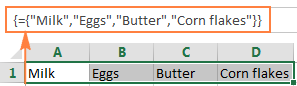What you have just done is create a one-dimensional horizontal array. Nothing dreadful so far, right?

## What is an array formula in Excel?

The difference between an array formula and a regular formula is that an array formula processes several values instead of just one. In other words, an array formula in Excel evaluates all individual values in an array and performs multiple calculations on one or several items according to the conditions expressed in the formula.

Not only can an array formula deal with several values simultaneously, it can also return several values at a time. So, the results returned by an array formula is also an array.

Array formulas are available in all versions of Excel 2019, Excel 2016, Excel 2013, Excel 2010, Excel 2007 and lower.

And now, it seems to be the right time for you to create your first array formula.

#### Simple example of Excel array formula

Suppose you have some items in column B, their prices in column C, and you want to calculate the grand total of all sales.

Of course, nothing prevents you from calculating subtotals in each row first with something as simple as `=B2*C2` and then sum those values: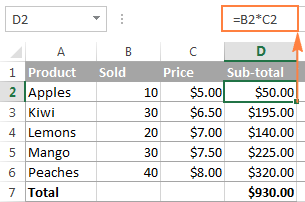However, an array formula can spare you those extra key strokes since it gets Excel to store intermediate results in memory rather than in an additional column. So, all it takes is a single array formula and 2 quick steps:

1. Select an empty cell and enter the following formula in it:

`=SUM(B2:B6*C2:C6)`

2. Press the keyboard shortcut CTRL + SHIFT + ENTER to complete the array formula.

Once you do this, Microsoft Excel surrounds the formula with {curly braces}, which is a visual indication of an array formula.

What the formula does is multiply the values in each individual row of the specified array (cells B2 through C6), add the sub-totals together, and output the grand total: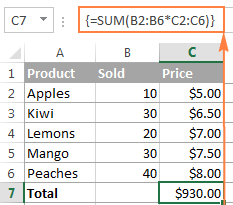This simple example shows how powerful an array formula can be. When working with hundreds and thousands of rows of data, just think how much time you can save by entering one array formula in a single cell.

#### Why use array formulas in Excel?

Excel array formulas are the handiest tool to perform sophisticated calculations and do complex tasks. A single array formula can replace literally hundreds of usual formulas. Array formulas are very good for tasks such as:

## How to enter array formula in Excel (Ctrl + Shift + Enter)

As you already know, the combination of the 3 keys CTRL + SHIFT + ENTER is a magic touch that turns a regular formula into an array formula.

When entering an array formula in Excel, there are 4 important things to keep in mind:

1. Once you've finished typing the formula and simultaneously pressed the keys CTRL SHIFT ENTER, Excel automatically encloses the formula between {curly braces}. When you select such a cell(s), you can see the braces in the formula bar, which gives you a clue that an array formula is in there.
2. Manually typing the braces around a formula won't work. You must press the Ctrl+Shift+Enter shortcut to complete an array formula.
3. Every time you edit an array formula, the braces disappear and you must press Ctrl+Shift+Enter again to save the changes.
4. If you forget to press Ctrl+Shift+Enter, your formula will behave like a usual formula and process only the first value(s) in the specified array(s).

Because all Excel array formulas require pressing Ctrl + Shift + Enter, they are sometimes called CSE formulas.

## Use the F9 key to evaluate portions of an array formula

When working with array formulas in Excel, you can observe how they calculate and store their items (internal arrays) to display the final result you see in a cell. To do this, select one or several arguments within a function's parentheses, and then press the F9 key. To exit the formula evaluation mode, press the Esc key.

In the above example, to see the sub-totals of all products, you select B2:B6*C2:C6, press F9 and get the following result.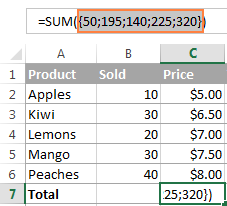Note. Please pay attention that you must select some part of the formula prior to pressing F9, otherwise the F9 key will simply replace your formula with the calculated value(s).

## Single-cell and multi-cell array formulas in Excel

Excel array formula can return a result in a single cell or in multiple cells. An array formula entered in a range of cells is called a multi-cell formula. An array formula residing in a single cell is called a single-cell formula.

There exist a few Excel array functions that are designed to return multi-cell arrays, for example TRANSPOSE, TREND, FREQUENCY, LINEST, etc.

Other functions, such as SUM, AVERAGE, AGGREGATE, MAX, MIN, can calculate array expressions when entered into a single cell by using Ctrl + Shift + Enter.

The following examples demonstrate how to use a single-cell and multi-cell array formula.

#### Example 1. A single-cell array formula

Suppose you have two columns listing the number of items sold in 2 different months, say columns B and C, and you want to find the maximum sales increase.

Normally, you would add an additional column, say column D, that calculates the sales change for each product using a formula like `=C2-B2`, and then find the maximum value in that additional column `=MAX(D:D)`.

An array formula does not need an additional column since it perfectly stores intermediate results in memory. So, you just enter the following formula and press Ctrl + Shift + Enter:

`=MAX(C2:C6-B2:B6)`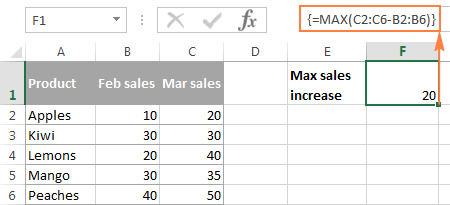#### Example 2. A multi-cell array formula in Excel

In the previous SUM example, suppose you have to pay 10% tax from each sale and you want to calculate the tax amount for each product with one formula.

Select the range of empty cells, say D2:D6, and enter the following formula in the formula bar:

`=B2:B6 * C2:C6 * 0.1`

Once you press Ctrl + Shift + Enter, Excel will place an instance of your array formula in each cell of the selected range, and you will get the following result: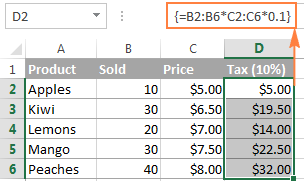#### Example 3. Using an Excel array function to return a multi-cell array

As already mentioned, Microsoft Excel provides a few so called "array functions" that are specially designed to work with multi-cell arrays. TRANSPOSE is one of such functions and we are going to utilize it to transpose the above table, i.e. convert rows to columns.

1. Select an empty range of cells where you want to output the transposed table. Since we are converting rows to columns, be sure to select the same number of rows and columns as your source table has columns and rows, respectively. In this example, we are selecting 6 columns and 4 rows.
2. Press F2 to enter the edit mode.
3. Enter the formula and press Ctrl + Shift + Enter.

In our example, the formula is:

`=TRANSPOSE(\$A\$1:\$D\$6)`

The result is going to look similar to this: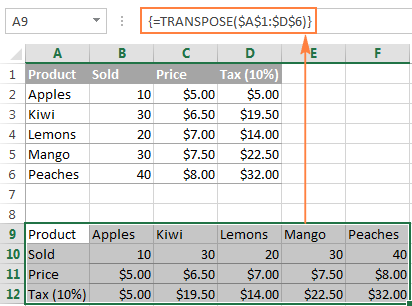This is how you use TRANSPOSE as a CSE array formula in Excel 2019 and earlier. In Dynamic Array Excel, this also works as a regular formula. To learn other ways to transpose in Excel, please check out this tutorial: How to switch columns and rows in Excel.

#### How to work with multi-cell array formulas

When working with multi-cell array formulas in Excel, be sure to follow these rules to get the correct results:

1. Select the range of cells where you want to output the results before entering the formula.
2. To delete a multi-cell array formula, either select all the cells containing it and press DELETE, or select the entire formula in the formula bar, press DELETE, and then press Ctrl + Shift + Enter.
3. You cannot edit or move the contents of an individual cell in an array formula, nor can you insert new cells into or delete existing cells from a multi-cell array formula. Whenever you try doing this, Microsoft Excel will throw the warning "You cannot change part of an array".
4. To shrink an array formula, i.e. to apply it to fewer cells, you need to delete the existing formula first and then enter a new one.
5. To expand an array formula, i.e. apply it to more cells, select all cells containing the current formula plus empty cells where you want to have it, press F2 to switch to the edit mode, adjust the references in the formula and press Ctrl + Shift + Enter to update it.
6. You cannot use multi-cell array formulas in Excel tables.
7. You should enter a multi-cell array formula in a range of cells of the same size as the resulting array returned by the formula. If your Excel array formula produces an array larger than the selected range, the excess values won't appear on the worksheet. If an array returned by the formula is smaller than the selected range, #N/A errors will appear in extra cells.

If your formula may return an array with a variable number of elements, enter it in a range equal to or larger than the maximum array returned by the formula and wrap your formula in the IFERROR function, as demonstrated in this example.

## Excel array constants

In Microsoft Excel, an array constant is simply a set of static values. These values never change when you copy a formula to other cells or values.

You already saw an example of an array constant created from a grocery list in the very beginning of this tutorial. Now, let's see what other array types exist and how you create them.

There exist 3 types of array constants:

#### 1. Horizontal array constant

A horizontal array constant resides in a row. To create a row array constant, type the values separated by commas and enclose then in braces, for example {1,2,3,4}.

Note. When creating an array constant, you should type the opening and closing braces manually.

To enter a horizontal array in a spreadsheet, select the corresponding number of blank cells in a row, type the formula `={1,2,3,4}` in the formula bar, and press Ctrl + Shift + Enter. The result will be similar to this: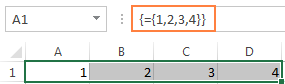As you see in the screenshot, Excel wraps an array constant in another set of braces, exactly like it does when you are entering an array formula.

#### 2. Vertical array constant

A vertical array constant resides in a column. You create it in the same way as a horizontal array with the only difference that you delimit the items with semicolons, for example:

`={11; 22; 33; 44}`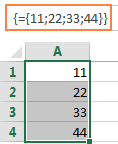#### 3. Two-dimensional array constant

To create a two-dimensional array, you separate each row by a semicolon and each column of data by a comma.

`={"a", "b", "c"; 1, 2, 3}`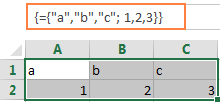### Working with Excel array constants

Array constants are one of the cornerstones of an Excel array formula. The following information and tips might help you use them in the most efficient way.

1. Elements of an array constant

An array constant can contain numbers, text values, Booleans (TRUE and FALSE) and error values, separated by commas or semicolons.

You can enter a numerical value as an integer, decimal, or in scientific notation. If you use text values, they should be surrounded in double quotes (") like in any Excel formula.

An array constant cannot include other arrays, cell references, ranges, dates, defined names, formulas, or functions.

2. Naming array constants

To make an array constant easier to use, give it a name:

• Switch to the Formulas tab > Defined Names group and click Define Name. Alternatively, press Ctrl + F3 and click New.
• Type the name in the Name
• In the Refers to box, enter the items of your array constant surrounded in braces with the preceding equality sign (=). For example:

`={"Su", "Mo", "Tu", "We", "Th", "Fr", "Sa"}`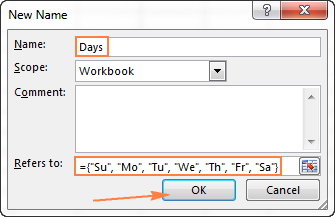• Click OK to save your named array and close the window.

To enter the named array constant in a sheet, select as many cells in a row or column as there are items in your array, type the array's name in the formula bar preceded with the = sign and press Ctrl + Shift + Enter.

The result should resemble this: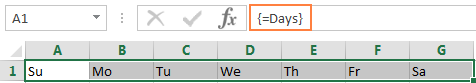3. Preventing errors

If your array constant does not work correctly, check for the following problems:

• Delimit the elements of your array constant with the proper character - comma in horizontal array constants and semicolon in vertical ones.
• Selected a range of cells that exactly matches the number of items in your array constant. If you select more cells, each extra cell will have the #N/A error. If you select fewer cells, only a part of the array will be inserted.

### Using array constants in Excel formulas

Now that you are familiar with the concept of array constants, let's see how you can use arrays informulas to solve your practical tasks.

#### Example 1. Sum N largest / smallest numbers in a range

You start by creating a vertical array constant containing as many numbers as you want to sum. For example, if you want to add up 3 smallest or largest numbers in a range, the array constant is {1,2,3}.

Then, you take either LARGE or SMALL function, specify entire range of cells in the first parameter and include the array constant in the second. Finally, embed it in the SUM function, like this:

Sum the largest 3 numbers: `=SUM(LARGE(range, {1,2,3}))`

Sum the smallest 3 numbers: `=SUM(SMALL(range, {1,2,3}))`

Don't forget to press Ctrl + Shift + Enter since you are entering an array formula, and you will get the following result: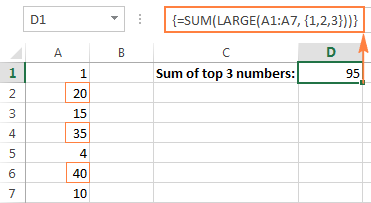In a similar fashion, you can calculate the average of N smallest or largest values in a range:

Average of the top 3 numbers: `=AVERAGE(LARGE(range, {1,2,3}))`

Average of the bottom 3 numbers: `=AVERAGE(SMALL(range, {1,2,3}))`

#### Example 2. Array formula to count cells with multiple conditions

Suppose, you have a list of orders and you want to know how many times a given seller has sold given products.

The easiest way would be using a COUNTIFS formula with multiple conditions. However, if you want to include many products, your COUNTIFS formula may grow too big in size. To make it more compact, you can use COUNTIFS together with SUM and include an array constant in one or several arguments, for example:

`=SUM(COUNTIFS(range1, "criteria1", range2, {"criteria1", "criteria2"}))`

The real formula may look as follows:

`=SUM(COUNTIFS(B2:B9, "sally", C2:C9, {"apples", "lemons"}))`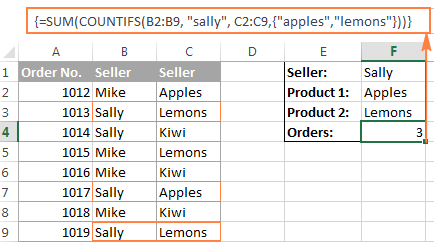Our sample array consists of only two elements since the goal is to demonstrate the approach. In your real array formulas, you may include as many elements as your business logic requires, provided that the total length of the formula does not exceed 8,192 characters in Excel 2019 - 2007 (1,024 characters in Excel 2003 and lower) and your computer is powerful enough to process large arrays. Please see the limitations of array formulas for more details.

And here is an advanced array formula example that finds the sum of all matching values in a table: SUM and VLOOKUP with an array constant.

## AND and OR operators in Excel array formulas

An array operator tells the formula how you want to process the arrays - using AND or OR logic.

• AND operator is the asterisk (*) which is the multiplication symbol. It instructs Excel to return TRUE if ALL of the conditions evaluate to TRUE.
• OR operator is the plus sign (+). It returns TRUE if ANY of the conditions in a given expression evaluates to TRUE.

#### Array formula with the AND operator

In this example, we find the sum of sales where the sales person is Mike AND the product is Apples:

`=SUM((A2:A9="Mike") * (B2:B9="Apples") * (C2:C9))`

Or

`=SUM(IF(((A2:A9="Mike") * (B2:B9="Apples")), (C2:C9)))`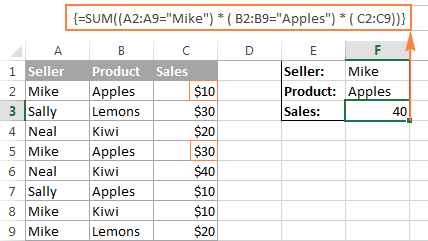Technically, this formula multiplies the elements of the three arrays in the same positions. The first two arrays are represented by TRUE and FALSE values which are the results of comparing A2:A9 to Mike" and B2:B9 to "Apples". The third array contains the sales numbers from the range C2:C9. Like any math operation, multiplication converts TRUE and FALSE to 1 and 0, respectively. And because multiplying by 0 always gives zero, the resulting array has 0 when either or both conditions are not met. If both conditions are met, the corresponding element from the third array gets into the final array (e.g. 1*1*C2 = 10). So, the result of multiplication is this array: {10;0;0;30;0;0;0;0}. Finally, the SUM function adds up the array's elements and return a result of 40.

#### Excel array formula with the OR operator

The following array formula with the OR operator (+) adds up all sales where the sales person is Mike OR product is Apples:

`=SUM(IF(((A2:A9="Mike") + (B2:B9="Apples")), (C2:C9)))`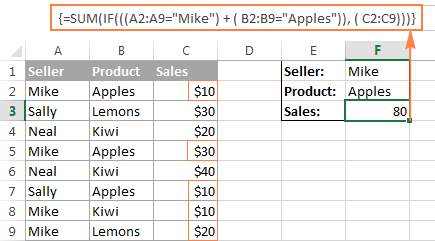In this formula, you add up the elements of the first two arrays (which are the conditions you want to test), and get TRUE (>0) if at least one condition evaluates to TRUE; FALSE (0) when all the conditions evaluates to FALSE. Then, IF checks if the result of addition is greater than 0, and if it is, SUM adds up a corresponding element of the third array (C2:C9).

Tip. In modern versions of Excel, there is no need to use an array formula for this kind of tasks - a simple SUMIFS formula handles them perfectly. Nevertheless, the AND and OR operators in array formulas may prove helpful in more complex scenarios, let alone a very good gymnastics of mind : )

## Double unary operator in Excel array formulas

If you've ever worked with array formulas in Excel, chances are you came across a few ones containing a double dash (--) and you may have wondered what it was used for.

A double dash, which is technically called the double unary operator, is used to convert non-numeric Boolean values (TRUE / FALSE) returned by some expressions into 1 and 0 that an array function can understand.

The following example will hopefully make things easier to understand. Suppose you have a list of dates in column A and you want to know how many dates occur in January, regardless of the year.

The following formula will work a treat:

`=SUM(--(MONTH(A2:A10)=1))`

Since this is an Excel array formula, remember to press Ctrl + Shift + Enter to complete it.

If you are interested in some other month, replace 1 with a corresponding number. For example, 2 stands for February, 3 means March, and so on. To make the formula more flexible, you can specify the month number in some cell, like demonstrated in the screenshot: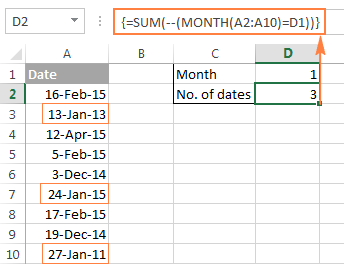And now, let's analyze how this array formula works. The MONTH function returns the month of each date in cells A2 through A10 represented by a serial number, which producing the array {2;1;4;2;12;1;2;12;1}.

After that, each element of the array is compared to the value in cell D1, which is number 1 in this example. The result of this comparison is an array of Boolean values TRUE and FALSE. As you remember, you can select a certain portion of an array formula and press F9 to see what that part equates to: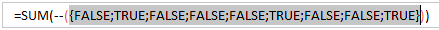Finally, you have to convert these Boolean values to 1's and 0's that the SUM function can understand. And this is what the double unary operator is needed for. The first unary coerces TRUE/FALSE to -1/0, respectively. The second unary negates the values, i.e. reverses the sign, turning them into +1 and 0, which most of Excel functions can understand and work with. If you remove the double unary from the above formula, it won't work.

I am hopeful this short tutorial has proved helpful on your road to mastering Excel array formulas. Next week, we are going to continue with Excel arrays by focusing on advanced formula examples. Please stay tuned and thank you for reading!

## You may also be interested in

1. Good day. Can some assist. which function is used to auto fill these sheet on the dates side. I need short cut on my excel to auto fill the dates like that I only know control D which is time consuming.
25-Oct-17 Stopped 1203
25-Oct-17 Stopped 1203
25-Oct-17 Stopped 1203
25-Oct-17 Stopped 1203
26-Oct-17 Stopped 1203
26-Oct-17 Stopped 1203
26-Oct-17 Stopped 1203
26-Oct-17 Stopped 1203
27-Oct-17 Stopped 1203
Stopped 1203
Stopped 1203
Stopped 1203
28-Oct-17 Stopped 1203
Stopped 1203
Stopped 1203
Stopped 1203
Stopped 1203
29-Oct-17 Stopped 1203
Stopped 18
Stopped 3001
Stopped 3010
30-Oct-17 Stopped 18
Stopped 3001
Stopped 3010
Stopped 3011
Stopped 3012

• Hello,

We have another article that describes how to fill empty cells in your table. Please have a look at it.

2. Hi Sir,
I have a data base, It includes columns A to J. From A- Name, Item Ref: Product, Location, Qty, Delivered Qty, Request date, Promised Date, etc.

Under Name there are more customer names and one customer name has more than one records.
Then how can I get displayed all the records related to a customer in a different sheet.
Ex. If I type the customer name Ann in a different sheet, then I need to see all the records available under Ann.
So what is the formula that helps me.

Thanks,
Udaya.

3. There is a mistake made in the post. The * is not the logical AND operator when used in array functions. It is simply that the Boolean values when used in arithmetic operators are automatically converted to numeric equivalents. So the section on AND and OR functions is wrong. Take for example the following formula presented in that section:
=SUM((A2:A9="Mike") * ( B2:B9="Apples") * ( C2:C9))

The "A2:A9="Mike"" returns a Boolean which is then converted to a numeric when it is multiplied with "B2:B9="Apples"" which in return is multiplied to "C2:C9". When both "A2:A9="Mike"" and "B2:B9="Apples"" are true, the corresponding value from "C2:C9" is taken into the sum calculation. The expression looks as follows 1*1*C.

• Hello Kuze,

It is true that when the logical values of TRUE and FALSE are used in arithmetic operations, they are converted to 1 and 0, respectively. But it is also true that in array formulas, multiplication acts as the logical AND function because it follows the same rules as the AND operator. That is, multiplication returns TRUE (1) only when all of the elements are TRUE. If any of the elements is FALSE (0), the result is FALSE (because multiplying by 0 always gives zero).

On the other hand, addition acts as the OR operator because it returns TRUE (>0) if any of the elements is TRUE. And the result is FALSE (0) only when all elements are FALSE.

4. I am just not grasping how to get the formula I want, though I know the answer is in there. I want to have a formula look up a specific item (say 'sam's apples) and then fill in the corresponding rows with the last year's sales by month. In other words (currently):
a b c d e
Company Jan Feb March April
1 Sam's apples

2

3

What I want once it pulls the data from a separate spreadsheet:

a b c d e
Company Jan Feb March April
1 Sam's apples \$532 \$225 \$632 \$1032

2

3

I have over 2000 rows to sort and the company and the sales \$ do not match up. Thanks ahead-

5. i want to create this type of table to sort the data
col A B c
type weight colD E F G H
Gr-1 Gr2 1000 Gr1 Gr2 Gr3 Gr4 up to Gr17
Gr2 Gr3 2000 Gr1 1000 4000
Gr3 Gr4 3000 Gr2 2000
Gr1 Gr3 4000 Gr3 3000

6. Светлана, спасибо за такую развернутую статью, наконец-то исчерпывающее объяснение про формулы массивов!

7. I need to display name of students separated with Comma in one cell holding same grade.

8. Hello everyone!
Dear Svetlana, how to Sum numbers from array of text cells? textNUMBERtext A1:D100

This working with single cell: =LOOKUP(99^99;--("0"&MID(A1;MIN(SEARCH({0\1\2\3\4\5\6\7\8\9};A1&"0123456789"));ROW(\$1:\$1))))

={OFFSET(INDIRECT("'GEN rates'!E"&MATCH(RIGHT(tool!K23,2)&A2&tool!F21,'GEN rates'!A68:A79&'GEN rates'!B68:B79&'GEN rates'!C68:C79,0)),ROW(\$A\$68)-1,0)*(tool!M23-tool!H23)+OFFSET(INDIRECT("'GEN rates'!E"&MATCH(RIGHT(tool!K23,2)&A2&tool!H21,'GEN rates'!A68:A79&'GEN rates'!B68:B79&'GEN rates'!C68:C79,0)),ROW(\$A\$68)-1,0)*(tool!M23-tool!F23)+IF(RIGHT(tool!K23,2)="EU",(tool!G23+tool!I23)*'GEN rates'!E80,0)}

while the following one with nested IF returns Value# error?

={IF('GT support'!B11<3,OFFSET(INDIRECT("'GEN rates'!E"&MATCH(RIGHT(tool!K23,2)&A2&tool!F21,'GEN rates'!A68:A79&'GEN rates'!B68:B79&'GEN rates'!C68:C79,0)),ROW(\$A\$68)-1,0)*(tool!M23-tool!H23)+OFFSET(INDIRECT("'GEN rates'!E"&MATCH(RIGHT(tool!K23,2)&A2&tool!H21,'GEN rates'!A68:A79&'GEN rates'!B68:B79&'GEN rates'!C68:C79,0)),ROW(\$A\$68)-1,0)*(tool!M23-tool!F23)+IF(RIGHT(tool!K23,2)="EU",(tool!G23+tool!I23)*'GEN rates'!E80,0),"incl.in FOB")}

I have been racking my brains to no avail so far, and seek any kind of advice to make the array formula work under the additional IF condition in the second case (otherwise the formulas are the same)

10. how to give an array as input?

• just name a range, and use the range name as input.

11. In the first example you say the grand total can be calculated with "=SUM(B2:B6*C2:C6)" but this should actually be "=SUMPRODUCT(B2:B6*C2:C6)"

• Hi Dutch,

This can actually be either, array SUM or SUMPRODUCT, which is an array formula by its nature.

12. Hello,

I need some help with calculating percentiles while using an array formula. Current formula is:

=IF(B7:B15>6,PERCENTILE(IF(Data!\$G:\$G=ReportPages!A1,IF(Data!\$E:\$E=ReportPages!A7:A15,IF(Data!\$I:\$I>0,Data!\$I:\$I))),0.1),"*")
ENTER +CTRL + SHIFT

The return value is #N/A

I isolated the issue to the "ReportPages!A7:A15" portion of the formula. It works fine with I change this to reference a single cell. Any help would be great.

13. Hi Svetlana;

Think you for your efforts to make learn Excel tools.I would like to Know if i can put for example {1; 2; 3} in the rows of the Offset function,and what it happened if i had put it and used all formula in sum function.

Best Regart

14. Wow!

Am in love with your articles. Very easy to understand.

I dreaded working with arrays initially.

Thanks for putting a smile on my face:-)

15. i have data in that i need to lookup 2 values matching data should on third cell and its duplicate data, suppose the data like

DATA
Product mm/dd/yyyy mm/dd/yyyy Sales
apples 11/10/2015 11/11/2016 20
oranges 11/10/2015 11/11/2016 52
banana 11/10/2015 11/11/2016 35
apples 11/10/2013 11/9/2015 66
oranges 11/10/2013 11/9/2015 69
oranges 9/8/2012 10/10/2013 55

Result data would be below

Product Date Required result
Apples 11/11/2014 66
Orange 9/9/2013 55

16. I am currently doing a project in excel, it is a database for medicine with a running lot no. which means a lagundi table can have many lot no. w/c is based on the date it is produced, now i want to display in the sheet what are the list lagundi with a specific lot no. which first entered the warehouse that has a quantity availble so it is easy to locate? WHAT WILL I DO WHAT FORMULA, HELP ME PLEASE IF I CANT SUBMIT THIS IT IS A FAILING GRADE THAT AWAITS ME. THANK YOU

17. You are great and as a student of data analysis,I just joined your column by accident and i am enjoying every bit of information you have published in this field.
However, I want to know the real difference between using SUMIFS, COUNTIFS, and AVERAGEIFS to summarize data using multi-criteria and the INDEX+ MATCH COMBINATION.
Thanks.

18. hi dear,
i have one issue kindly help me
i have 2 row
suppose that
10 20 30 40 50 60 70 80 90
15 25 35 45 55 65 75 85 95
greater than formula???
if i put the value in cell (30) and i wanna search the greater value in 2nd row.Which formula i put in cell than show the answer is (35)
because 1st row 30 is greater than 2nd row 35.

19. i have a table.
coloumn names( employee name, basic salary, allowance, netsalary)
row names(employee 01, employee 02,.......,employee 10)

i want to extract the employee whose basic salary is grater than \$200.

how to do it?
i don't like use a filter method.

I linked a master sheet (some range of cells) with many other sheets (with the same cell reference range) using arrays. However, some functions are not working in the Master sheet. For eg. sum function does not work out within the cell ranges which are linked. Any ideas????

21. thanks you all, for puting someone into light

22. I have a list of potential % changes in a variable and another list with the probability that % change will occur. If I use the Data Analysis Random Number Generator it does not allow the number to be recalculated and accept changes in the simulation. How do I generate a non-static random % change based on the assigned probabilities?

23. Hi, need help comparing text (separated by commas) in 2 cells, to see what is different. For example:

A1 = John, Matt, Shelly
A2 = Polly, Shelly

We can see that John and Matt have been removed from A2, however Polly has been added. What is the best way to go about pinpointing the differences between two cells?

24. Hi, need help comparing text (separated by commas) in 2 cells, to see what is different. For example:

A1 = John, Matt, Shelly
A2 = Polly, Shelly

We can see that John and Matt have been removed from A2, however Polly has been added. What is the best way to go about pinpointing the differences between two cells?

25. i have a doubt how can we convert a cell that contains a range in to many rows of whole number
demo
3-6 range has to be converted to 3
4
5
6

26. Need Help

Hi am trying to use vlookup to extract muitiple column details for multiple id using array concept but am just able to extract the 1st required column details.

Here is the formula:
{=VLOOKUP(I3:I11,\$A\$1:\$G\$278,{2,3,4,5},0)}

help me to identify where am going wrong

• Hi amrutha,

27. Hi
i nee to know if a value of array P
1-Jan-15
3-Abr-15
25-Abr-15
1-Mai-15
10-Jun-15
15-Ago-15
8-Dez-15
25-Dez-15

exist in array AK.
01-01-2015 20:00
01-01-2015 23:00
02-01-2015 00:00
02-01-2015 01:00
02-04-2015 23:00
03-04-2015 00:00
03-04-2015 01:00
24-04-2015 23:00
25-04-2015 00:00
25-04-2015 01:00
30-04-2015 23:00
01-05-2015 00:00
01-05-2015 01:00

if exist than indicate with a "D". I tried with the expression
={IF(INT(VALUE(AP10))=(VALUE(\$AK\$10:\$AK\$17));"D";"xxx")}
but this does not works. can you help? thanks

• Hi Joao Barros,

You should use the following array formula:
{=IF(SUM(IF(TEXT(\$B\$1:\$B\$10,"yyyy-mm-dd")=TEXT(A1,"yyyy-mm-dd"), 1, 0)), "D", "xxx")}
Where the array AK is in B1:B10 and the array P is in A1:A10.

28. HI,
MY QUESTION IS ,I CREATE A1 TO A12 MULTIPLE IN RESULT OF B1 TO B12 IN ALL CELL FROM MULTIPLE VALUE OF *3. PLEASE PROVIDE INFORMATION.
THANKS

• Hi CHANDRAPAL SINGH LODHI,

29. I have a list of (+) and (-) in a column, each one needs to be assigned a number based on it's position to the last of it's kind on the list. So the list may have 2 (-) then 3 (+) then another 2 (-). I'd like to run a formula that will assign an odd number to the (-) and an even number to (+) but consecutively. So the first two would be 1,3, the next three would be 2,4,6, then the next two would be 5,7. I've tried setting to columns with the numbers and using an array formula({=if(A1:A8="(-),C1:C8,D1:D8)}, assuming C column has 1,3,5,7,9,11,13 and the D column has 2,4,6,8,10,12,14 listed) the problem I've come across is that it takes it consecutively for the last number so it would go 1,3,6,8,10,11,13 instead of the desired 1,3,2,4,6,5,7. Any ideas how to fix this?

• Hi Krista,

If I understand you correctly you should use the following array formula:

{=IF(A1="-",SUM(IF(\$A\$1:\$A\$7="-",1*IF(ROW(\$A\$1:\$A\$7)<=ROW(A1),1,0),0)) *2 - 1, SUM(IF(\$A\$1:\$A\$7="+",1*IF(ROW(\$A\$1:\$A\$7)<=ROW(A1),1,0),0)) *2)}

Where the values "+" and "-" are in A1:A7.

30. 2 kg + 3 kg = 5 kg.

I want the kg should come once the calculation is completed as above eg. shows

31. Hi,

Please could you help with a formula.

I am trying to work out a moving average for the following:

Week Commencing Number Value
20/07/2015 7 £2,000.00
27/07/2015 37 £5,000.00
03/08/2015 73 £397,270.97
10/08/2015 40 £238,580.87

So for example week 20/07/2015 I'm using the formula =sum(d2/c2). I want a continuous formula that adds the weekly numbers as each week passes so for the second week the sum would be 7+37 =44 and the value would be £2,000 + 5,000 = 7,000 so £7,000/44.

I require the formula to be continuous as I have many weeks to work out the average.

Jason

32. Hi,

If the cell A1 is more the 45 to add 15 and if A1 is less than 45 to times/multiply by 1.2

Please if anyone could kindly help which formula i would have to use

Many Thanks,

Abe

• Hi Abe,

If my understanding of the task is right, you can use the following simple formula:

=IF(A1>45, A1+15, A1*1.2)

33. Can you please explain to me what causes the following inconsistency with a non-array formula?

=LARGE(IFERROR({4,5},0),1) returns 4

BUT when evaluating the IFERROR part using F9 the formula returns:

=LARGE(IFERROR({4,5},0),1)
[F9] =LARGE({4,5},1)
[enter] = 5

Please do not reply that the solution is to enter the formula using Ctrl+Shift+Enter, my problem is more subtle: why does Excel give a different result when the formula is evaluated in 2 steps?

34. Thanks a lot for this nice article which explains a lot.

35. Hi,
I've extracted data specific to employee names from a master sheet using array formula.

I'm struggling to create a dynamic chart to display this data using excel.

Any tips on how this can be done?

36. Is it possible to define an array that is a combination of cell ranges and constants? For example how would one use a a three element array made up of {A2, 2-A2, 1} in a formula where A2 is a cell reference? Seems like it should be easy, but I'm stumped. Thanks!

• I have entered this formula and got the correct result:
{=SUM(A1,2-A2, 1)}

37. I have an Excel dataset consisting of 500 rows by 7 columns. I am generating additional data points from this dataset. I need to multiply (or other function) each row by all 500 rows, creating 250,000 new rows of data. Each cell needs to function as a constant that is multiplied by all the other cells in the same column (which are not acting as constants). How do I do this efficiently?

https://support.ablebits.com/blog_samples/array-formulas-functions-excel_13_MultiplyEachRow.xlsx

Enter five values to A1: A5

Use the following formula to get the first multiplier address in 25 resulting rows:

Use this formula to get the second multiplier address:

To get a value using the address, please enter the INDIRECT function.

The final formula in rows A6: A31 will be as follows:

• That was brilliant, Fedor! I applied your example solution to my dataset and got exactly what I requested. Thanks!

Unfortunately, I found out that Excel (2007) can only handle 32,000 rows for graphing or statistical analysis. Now am I thinking of condensing the 250,000 rows generated with your formula down to 25,000 rows. This can be done by sorting the dataset, then averaging each successive 10 rows. I tried that, but I am getting a moving average rather than averaging 10 rows to make one new row, then averaging the next 10 rows to make the second new row, and so on.

Could you suggest an approach? I am sorry to trouble you again, but you are the maestro.

• I found a solution that works very well, which I will post in case it is useful to anyone else:
>>If your data starts from An (where n is 2,3,4,...), use this formula:
=AVERAGE(INDEX(A:A,n+10*(ROW()-ROW(\$B\$1))):INDEX(A:A,n-1+10*(ROW()-ROW(\$B\$1)+1))
where you should change n to 2,3,4,...

38. Unit COR SCM SMH
CODE 10101 10102 10103
COR 10101 - (411,876,279) 84,314,441
SCM 10102 411,906,311 - -
SMH 10103 (84,314,439) - -

Which formula works to check Difference between COR with SCM, COR with SMH,SCM with COR, SCM with SMH.

39. HI, I am currently studying Design & produce business documents.
I have an excel sheet with name of supplier, product name, unit price, unit sales and Total sales. I have been told to add an subtotal to this spreadsheet, go to subtotal, click on unit sales and total sales, tick replace current subtotals and Summary below data and apply. It gives me a message I can not change part of an array. How to I get around this? Many thanks Leanne Waldron

40. I've been using array formulas for 13 years and fear a recent (mandatory) upgrade to 2013 may have broken them. Until now, I could enter a formula like

=SUM((\$A:\$A=\$A2)*(\$B:\$B=\$B2)*(\$C:\$C)*(\$D:\$D))
{using cntrl-shift-enter, of course}
This would:
*Allow me to test on multiple conditions vs. columns A and B (etc.)
*Combine weighted elements across columns C and D (etc.)

This is simplified for illustration - test values were often fed from drop-downs, and both conditions and products often spanned numerous columns. All worked well, and the ability to specify an entire column made formula entry easy and flexible in the face of varying data counts. Arrays also offered advantages over pivot tables in always refreshing dynamically and offered more persistent & flexible formatting than the fairly rigid pivot table formatting options.

Now in Excel 2013, this array formula appears to break as text header rows evaluate to errors. Previously, headers led to false conditions equaling zero, and the sum continued to a correct answer. Now, I encounter errors when using the array format or an incorrect final 0 if entering as a non-array SumProduct function & syntax.

Are there any options to restore the old evaluation rules (False*Text = 0)? I could be precise in choosing only numeric ranges or rewrite as a set of dynamic named ranges, but that's considerable effort for a number of legacy workbooks.

• Newer Excel versions attempt to 'parse' the TEXT cell content as a numeric value before applying the '*' multiply operator, i.e. as if it was implicitly fed to a VALUE() function call and thus produce a #VALUE error for non-numeric strings.

The way to stop these errors from propagating into your SUM is to use IFERROR(), i.e.

{=SUM(IFERROR(\$A:\$A=\$A2, 0)*IFERROR(\$B:\$B=\$B2, 0)*(\$C:\$C)*(\$D:\$D))}

should work for you.

Since you use multiply and add operators as AND/OR 'replacements', it's better to write IFERROR(cond, 0) rather than IFERROR(cond, FALSE) as that 'FALSE' would have to be converted to a numeric zero(0) anyway.

Note that IFERROR will also filter out (and replace by FALSE=0) any NaN's produced by any calculations in the range (#DIV/0 errors, etc.) but I consider that a benefit here.

41. Many thanks for the valuable hints.
I'm trying array formulas with (dynamic)named ranges (column names) from an excel (formated) table and I do not get it to work.
Is it not possible or is there any trick for getting it?

• Im having the same issue. Trying to use array formulas to create ranges for an excel chart. It wont allow me to enter it directly in the chart range/s and when I create a named range using the array formula it doesnt work either. (I dont want to alter the original diplayed dataset or create an additional table using the array formula). Is this possible? Any help would be greatly appreciated!

• Hello Juan,

Please send us a workbook with the sample data and formulas to support@ablebits.com. We'll try to help.

42. Thanks for all the effort put into developing the tutorial. It write-up and examples and explanations are extremely clear and easy to understand.

43. I need a function to split the letters of a word in different cells vertically in ms excel
could you help me with this

• Hello!

I think you can use the LEFT and MID functions. For example:

Extract the 1st letter: =LEFT(A1, 1)

Extract the 2nd letter: =MID(A1, 2, 1)

Extract the 3rd letter: =MID(A1, 3, 1)

And so on.

44. I have a data with duplicate name in column A and seal no in column which are unique in nature, can you help me in getting the data horizontally with text in column C falling in vertical below the Names.

Branch Name Rec DateTime Seal_no
Vinay 18-05-15 15:08 j2437981
Vinay 18-05-15 15:12 j2437971
Vinay 18-05-15 15:19 J2416597
Vinay 18-05-15 15:21 J2454248
Vinay 18-05-15 15:23 j2435055
SANDRA 18-05-15 15:08 j2416440
SANDRA 18-05-15 15:12 j2437984
SANDRA 18-05-15 15:19 J2437293
SANDRA 18-05-15 15:21 j2435005
SANDRA 18-05-15 15:22 J2438075

Need in horizontal in excel

Vinay SANDRA
j2437981 18-05-15 15:08 j2416440 18-05-15 15:08
j2437971 18-05-15 15:12 j2437984 18-05-15 15:12
J2416597 18-05-15 15:19 J2437293 18-05-15 15:19
J2454248 18-05-15 15:21 j2435005 18-05-15 15:21
j2435055 18-05-15 15:23 J2438075 18-05-15 15:22

• Vinay,

If you want to place the data into separate worksheets based on the name, then please try out our Split Table Wizard.

If your task is to place all the data into different columns on one worksheet, then you need a VBA script. Sorry we can’t help you with this. Please try to find the solution on mrexcel.com/excelforum.com

45. Due to the fact that I use the European decimal settings (, for decimal and . for digit grouping) my List separator is ;
This means that I can only creat vertical arrays, because when I try to create horizontal arrays, for instance ={10,20} and then CTRL+SHIFT+ENTER, I get 10,2 in both cells instead of 10 in the first and 20 in the second.
How to solve this without changing my decimal setting to a dot?

• Hi Mike,

This seems to be a common international issue that many users struggle with : (

If comma is decimal symbol, then \ is used in place of comma in Array.

If semi colon is decimal symbol, then \ is used in place of semi colon in Array. (Semi colon is row separator)

46. I´m having problems with the transpose example and using formula debug to get a list of cell values.
Example:
formula is =transpose(A3:A15)
When I hit F9 the cell values are delimited by a \ instead of comma, like this:
={“Lebanon”\”Israel”\”Turkey”\”Estonia”\”Sweden”\”Greece”\”Tunisia”\”Russia”\”Middle East”\”Denmark”\”Spain”\”Belgium”\”Poland”}

Any idea how/where to change that so I actually get a list like this?
{"Lebanon","Israel","Turkey","Estonia","Sweden","Greece","Tunisia","Russia","Middle East","Denmark","Spain","Belgium","Poland"}

Using Excel 2013 with English (US) language pack installed on German Win8.1 .

• Hi Kristian,

Most likely the backslash (\) is set as a List separator in your Windows Regional settings. Please check Control Panel > Region and Language > Additional settings. When setting the comma as the List separator, be sure to select some other symbol as the Decimal separator.

47. Just ran across your blog the other day and it is really insightful. In reference to the "And"/"Or" operator section, is it similar to how you can use a sumproduct and sign to complete a shorter countif without an array?

ex.